Magnetism and Superconductivity in the Quaternary Borocarbides

Inna Vishik June 17, 2007

(Submitted as coursework for Applied Physics 273, Stanford University, Spring 2007)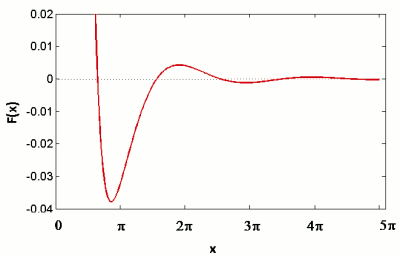Fig. 1: Spatial variation of RKKY susceptibility.

In most systems, the superconducting and magnetic ordered states are mutually exclusive. However, materials, including the quaternary borocarbides, coexistence can occur. The borocarbides, RT2B2C (R=rare earth, T=transition metal), crystallize for most members of the rare earth series, and they exhibit conventional BCS phonon-mediated superconductivity. They have generated interest in the decade since their discovery for two reasons: the superconducting transition temperature (Tc) is relatively high for a BCS superconductor, and the onset of magnetism occurs at temperatures close to Tc. The latter reason is particularly crucial, because it indicates similar energy scales for the two phenomena, making the borocarbides a good system to study the competition physics of two different ordering phenomena.

RKKY Exchange Interaction

The topic of rare earth magnetism is very rich and complicated, and this section is intended to serve as an introduction. Magnetic ordering in the borocarbides (and other rare earth compounds) is mediated by RKKY (Ruderman-Kittel-Kasuya-Yosida) exchange with the conduction electrons.  Consider the spin on an individual atom to be a magnet with a miniscule spatial extent. In the limit where the spatial extent is zero, the local magnetic field becomes a delta function, which can be expressed as fourier integral.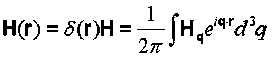where Hq=H. If we assume that the magnetization M is also a delta function, this implies that the electron gas responds completely to the perturbation of a magnetic ion: i.e. the susceptibility is a constant given by χ = Mq/Hq. However, the presence of a Fermi surface makes the electron gas less responsive for spatial frequencies q > kf, where kf is the Fermi wavevector. Thus, we must consider the frequency components of the susceptibility as well.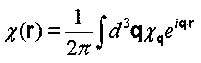χq is the response of an electron gas to a spatially varying magnetic field. Its value is found by perturbing the bloch wavefunctions of an electron gas to first order with the following periodic disturbance: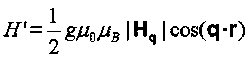The result of this calculation is quoted below: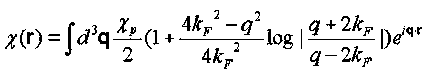Here, χp is the pauli susceptibility, given by: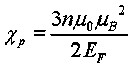Performing the integral leads to the following spatially-dependent susceptibility: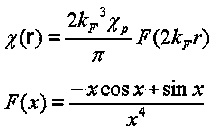This oscillatory susceptibility is plotted in Fig. 1. RKKY interaction allows for the ordering rare earth moments: one moment produces an oscillatory magnetization in its vicinity to which the moments of other atoms couple. The coupling can be ferromagnetic or antiferromagnetic, depending on the atomic distance.

de Gennes Scaling in the Borocarbides

The rare earth elements have the same valence, and often, as is the case for the nickel borocarbides, compounds will form for much of the series, providing a systematic variation of the magnetic properties. As we move to the left along the lanthanide series (Fig. 2), we see a roughly linear increase of TN, the antiferromagnetic transition temperature, with the de Gennes factor, and a concurrent suppression of Tc. The de Gennes factor (dG) is related to the Lande g factor and the total angular momentum, J, from Hund's rules, per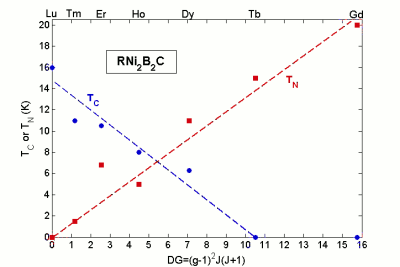Fig. 2: Tc and TN scale roughly linearly with the de Gennes factor (DG).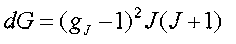The linear relation between the de Gennes factor and Tc and TN involves two parameters which are constant across the rare earth series: the strength of the exchange interaction with the local 4f moments (I) and the density of states at the Fermi level(N(εf)).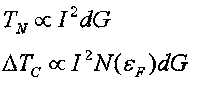This de Gennes scaling shows that both antiferromagnetism and suppression of superconductivity are governed by the same mechanism: exchange interaction with the 4f electrons. Furthermore, we see a competion between these two ordered states: as magnetism is strengthened, superconductivity is weakened. Next, we shall see how this interplay is evident as the system goes through magnetic phase transitions.

Case study: ErNi2B2C

This section will show some key experiments on ErNi2B2C, which beautifully illustrate the competition physics of the borocarbides. The crystal structure and the magnetic ordering is shown in Fig. 3. Although the crystal structure is the same for other borocarbides, these compounds exibit a variety of magnetic structures. In the Er compound, superconductivity sets in at 11K, and antiferromagnetism appears at 6K. In this ordered state, the spins lie in the a-b plane. Along the ordering wavevector, q, each spin is canted from the axis of its neighbor, creating a "spiral" ordered state. This ordering is incommensurate: the period of the spiral does not coincide with an integer number of lattice spacings. At 2.3 K, however, the periodicity becomes commensurate, and also develops a small ferromagnetic component.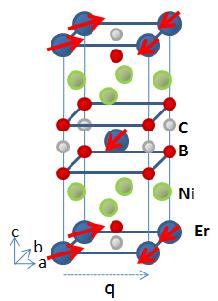Fig. 3: ErNi2B2C crystal structure. All the magnetism in the system comes from Er. Below 6 K, the Er spins order in an incommensurate spin density wave .

Two important parameters for characterizing a superconductor are the coherence length, ξ, (the "size" of a cooper pair) and the London penetration depth, λ (the distance which a DC magnetic field can penetrate into a superconductor). Small angle neutron scattering measurements showed that both of these quantities have an anomaly near TN , and those results are reproduced in Fig. 4.

One way of assessing the robustness of a superconducting state is by how readily it is destroyed by a magnetic field. There are two fields to consider: the lower critical field (Hc1) where quantized flux first enters a type II superconductor and the upper critical field (Hc2) where superconductivity is destroyed. These two quantities can be calculated from x and l using the following relations: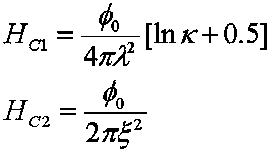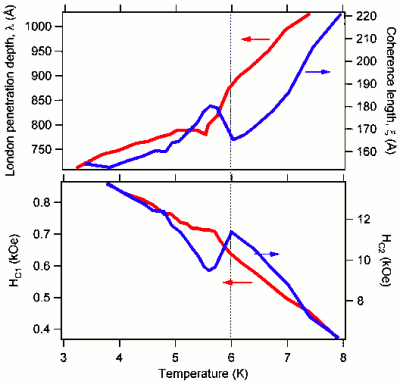Fig. 4: (top) Penetration depth and coherence length as determined from neutron scattering. (bottom) Upper and lower critical field, as determined from data in top panel. Vertical dotted line denoted TN. (From .)

Where φ0 is the superconducting flux quantum and κ = λ/ ξ. These quantities also show a kink near the magnetic phase transition. The dip in Hc2 demonstrates that superconductivity is weakest near TN—a property that is attributed to fluctuations associated with the phase transition. However, at lower temperatures, neither of the critical fields recover to values they would have had in the absence of long-range antiferromagnetic order, indicating that the magnetism in the system compromises the robustness of the superconducting state.

The possibility for antiferromagnetism to coexist with superconductivity is not entirely surprising because the net magnetism of this order is zero: as long as the coherence length, is larger than the spacing between the magnetic ions, the cooper pairs do not see a net magnetic field. However, the transition to weak ferromagnetism below 2.3K in ErNi2B2C is more surprising. The London equations predict that a uniform magnetic field is exponentially suppressed inside a superconductor, and even in type II superconductors which permit magnetic flux, the field is exponentially suppressed outside the flux tubes. In general, either the magnetic phase or the superconducting phase must be altered to permit coexistence, and in ErNi2B2C, it appears that the magnetic phase is altered to be more amenable to superconductivity. Several theories propose an oscillatory magnetic state which coexists with a uniform superconducting state below 2.3K, with a period smaller than the London penetration depth, and one such possibility is the Anderson-Suhl mechanism [2,4]. Since the borocarbides superconductors have singlet cooper pairs, the susceptibility of the electrons at long wavelengths drops in the superconducting state (bound pairs with net spin zero cannot respond to a DC magnetic field). The spin susceptibility does not recover until q~10 ξ-1, and the integral for determining the RKKY suscpetibility is altered to suppress the long wavelength (small q) contributions. The local maximum plotted in Fig. 1 shifts to a nonzero q, producing an oscillatory magnetization. Domains of oscillating magnetization were observed below 2.3K using scanning hall probe microscopy .

© 2007 Inna Vishik. The author grants permission to copy, distribute and display this work in unaltered form, with attribution to the author, for noncommercial purposes only. All other rights, including commercial rights, are reserved to the author.

References

 S. Blundell, Magnetism in Condensed Matter (Oxford, 2001).

 K-H Muller and V. N. Narozhnyi. Rep. Prog. Phys. 64, 943 (2001).

 P.L. Gammel et al., Phys. Rev. Lett. 82 1756 (1999).

 P.W. Anderson and H. Suhl. Phys. Rev. 116 898 (1959).

 H. Bluhm et al., Phys. Rev. B 73, 014514 (2006).